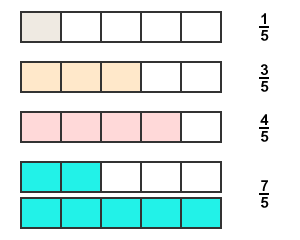# Like fractions

The fractions of equally divided different two or more whole quantities are called like fractions.

One whole quantity can be divided into numerous equal parts. If second whole quantity is also divided into same number of equal parts, the two whole quantities become like due to same division system. Therefore, the fractions of them are known as like fractions. The fraction of first whole quantity is like to the fraction of second whole quantity and vice-versa.

The concept of like fractions is not limited to two whole quantities but started with at least two quantities. Therefore, like fractions are the fractions which are considered from the two or more equally divided whole quantities.

## Example

Consider five rectangles and divide each rectangle into five equal parts.1. Select one part from total number of parts of first rectangle. So, the fraction of the selected portion is $\frac{1}{5}$
2. Select three parts from total number of parts of second rectangle. So, the fraction of the selected portion is $\frac{3}{5}$
3. In third rectangle, four parts are selected from total parts of the third rectangle. So, the fraction of selected parts is $\frac{4}{5}$
4. Assume, fourth and fifth rectangles form an improper fraction as a group. Two parts are selected from fourth rectangle and all parts are selected from fifth rectangle. Therefore, the fraction of this group is summation of fractions of both fourth and fifth rectangles. Therefore, the fraction of this group is $\frac{7}{5}$

According to this example, $\frac{1}{5}$, $\frac{3}{5}$, $\frac{4}{5}$ and $\frac{7}{5}$ are four fractions but all four fractions contain same denominator commonly and it is $5$. It is due to the division of all whole rectangles into equal number of divisions.

It is cleared that the fractions are like fractions if two or more fractions have same number in denominator.

Latest Math Topics
Latest Math Problems
Email subscription
Math Doubts is a best place to learn mathematics and from basics to advanced scientific level for students, teachers and researchers. Know more
Follow us on Social Media
###### Math Problems

Learn how to solve easy to difficult mathematics problems of all topics in various methods with step by step process and also maths questions for practising.

Learn more